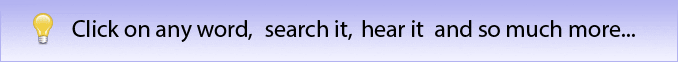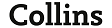# differential operator definition, differential operator meaning | English dictionary

Search also in: Web News Encyclopedia Images## differential operator

n   the mathematical operator del ∇, used in vector analysis, where ∇= i∂/∂x + j∂/∂y+ k∂/∂z, i, j, and k being unit vectors and ∂/∂bx, ∂/∂y, and ∂/∂z the partial derivatives of a function in x, y, and zdifferential
1    of, relating to, or using a difference
2    constituting a difference; distinguishing
3      (Maths)   of, containing, or involving one or more derivatives or differentials
4      (Physics, engineering)   relating to, operating on, or based on the difference between two opposing effects, motions, forces, etc.
differential amplifier
n
5    a factor that differentiates between two comparable things
6      (Maths)
a    an increment in a given function, expressed as the product of the derivative of that function and the corresponding increment in the independent variable
b    an increment in a given function of two or more variables, f(x1, x2, ...x>n), expressed as the sum of the products of each partial derivative and the increment in the corresponding variable.
7    an epicyclic gear train that permits two shafts to rotate at different speeds while being driven by a third shaft
8      (Chiefly Brit)   the difference between rates of pay for different types of labour, esp. when forming a pay structure within an industry
9    (in commerce) a difference in rates, esp. between comparable labour services or transportation routes

differential calculus
n   the branch of calculus concerned with the study, evaluation, and use of derivatives and differentials
Compare       integral calculus
differential coefficient
n     (Maths)      another name for       derivative
differential equation
n   an equation containing differentials or derivatives of a function of one independent variable. A partial differential equation results from a function of more than one variable
differential gear
n   the epicyclic gear mounted in the driving axle of a road vehicle that permits one driving wheel to rotate faster than the other, as when cornering
differential geometry
n   the application of differential calculus to geometrical problems
differential operator
n   the mathematical operator del ∇, used in vector analysis, where ∇= i∂/∂x + j∂/∂y+ k∂/∂z, i, j, and k being unit vectors and ∂/∂bx, ∂/∂y, and ∂/∂z the partial derivatives of a function in x, y, and z
differential windlass
n   a windlass employing the velocity ratio incurred in unwinding from a small drum while winding onto a larger drum rotating at a common speed,   (Also called)    Chinese windlass
wage differential
n   the difference in wages between workers with different skills in the same industry or between those with comparable skills in different industries or localities1